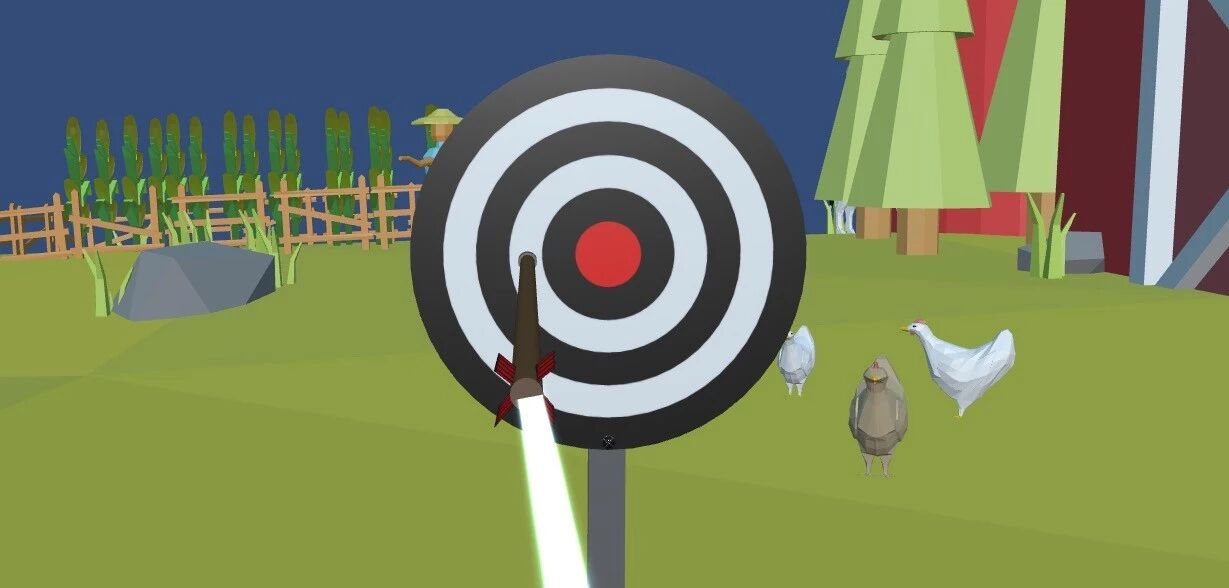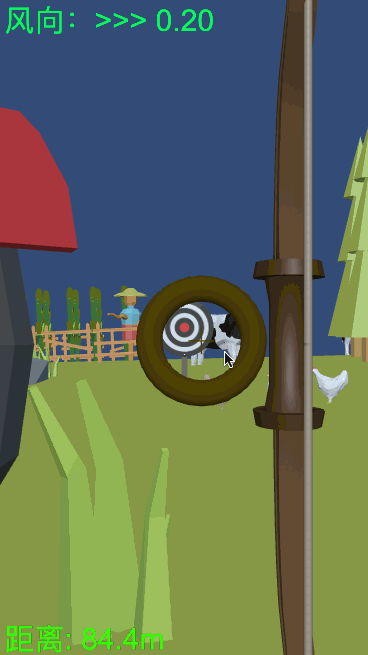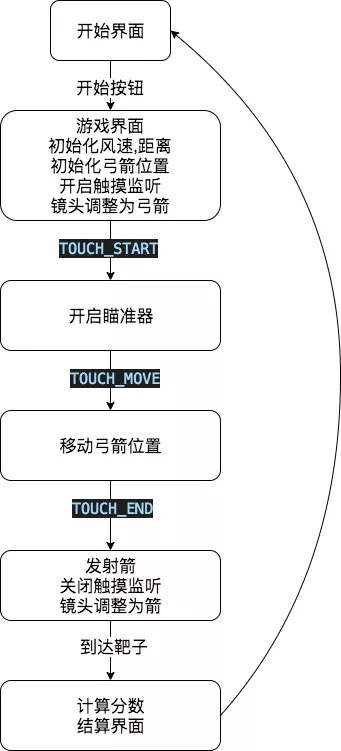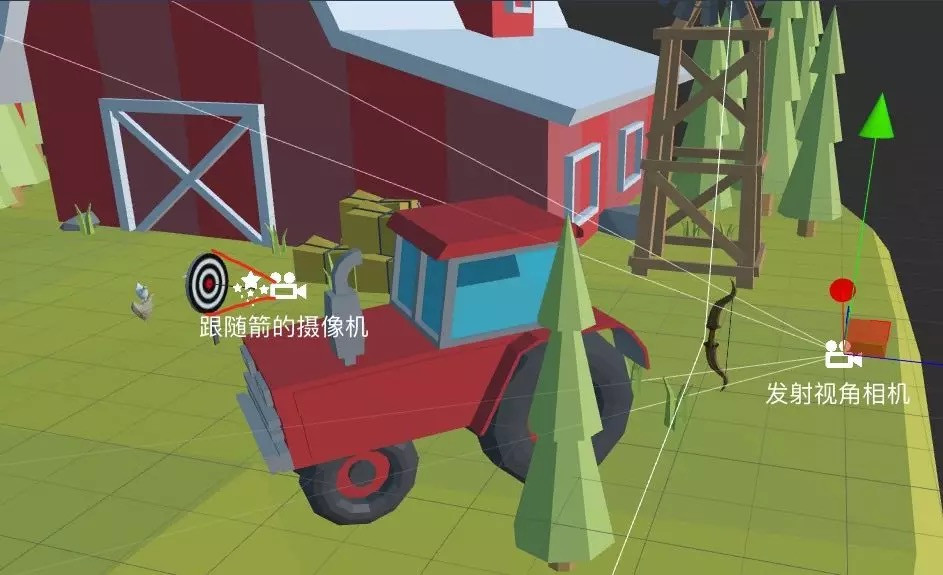# 置顶拇指射箭【代码分享】精选热门cocos creator 3d v1.0.0private onTouchMove(touch: Touch) {
const delta = touch.getDelta();
this.NodePos_bows.x -= delta.x * CONST_TOUCH_FACTOR;
this.NodePos_bows.y += delta.y * CONST_TOUCH_FACTOR;
}

export class NodePos extends Component {
private _curPos: Vec3 = cc.v3();
start() {
this._curPos = this.node.position;
}
get x() {
return this._curPos.x;
}
set x(x: number) {
this._curPos.x = x;
}
// 省略部分代码
update(deltaTime: number) {
this.node.position = this._curPos;
}
}

tweenUtil(this.NodePos_arrow)
.stop()
.to(5, { z: targetZ, x: targetX, y: targetY })
.to(1, {})
.call(() => {
this.gameOver();
})
.start()

const dis = this.NodePos_arrow.position.clone().subtract(this.NodePos_target.position).length();
const score = dis < CONST_TARGET_RADIUS ? ((1 - dis / CONST_TARGET_RADIUS) * 10).toFixed(2) : (‘0’);

【本文使用图片素材转载自Cocos社区作者：白玉无冰】